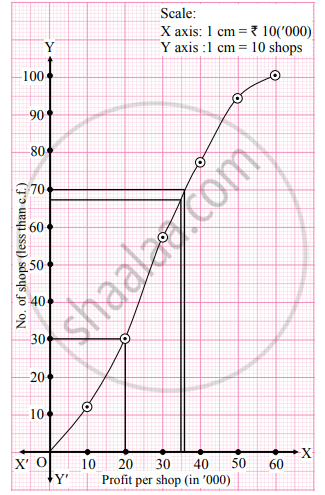# The following frequency distribution shows the profit (in ₹) of shops in a particular area of city: Profit per shop (in ‘000) No. of shops 0 – 10 12 10 – 20 18 20 – 30 27 30 – 40 20 40 – 50 17 50 - Mathematics and Statistics

Graph

The following frequency distribution shows the profit (in ₹) of shops in a particular area of city:

 Profit per shop (in ‘000) No. of shops 0 – 10 12 10 – 20 18 20 – 30 27 30 – 40 20 40 – 50 17 50 – 60 6

Find graphically The limits of middle 40% shops.

#### Solution

The less than cumulative frequency table is

 Profit per shop (in ‘000) No. of shops (f) CumulativeFrequency (less than type) 0 – 10 12 12 10 – 20 18 30 20 – 30 27 57 30 – 40 20 77 40 – 50 17 94 50 – 60 6 100 Total 100

Points to be plotted are (10, 12), (20, 30), (30, 57), (40, 77), (50, 94), (60, 100).The middle 40% shops will lie between the limits given by P30 and P70.
N = 100
For P30 (30"N")/(100)=(30(100))/(100) = 30

For P70 (70"N")/(100)=(70(100))/(100) = 70
∴ We take the points having Y co-ordinates 30 and 70 on Y-axis. From these points, we draw lines parallel to X-axis. From the points where these lines intersect the curve, we draw perpendiculars on X-axis.
X-Co-ordinates of these points gives the values of P30 and P70.
∴ P30 ≈ 20, P70 ≈ 36

Concept: Graphical Location of Partition Values
Is there an error in this question or solution?
Chapter 1: Partition Values - Exercise 1.3 [Page 19]

Share